# Twisty Puzzles

Twisty puzzles are puzzles that are inspired by Rubik's Cube.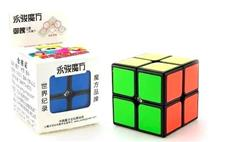2x2 Cube \$5.95 A good starting place for exploring the world of twisty puzzles, this simple cube is not as easy as it looks.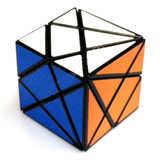Axis Cube \$8.95 This unique twisty puzzle is based on a complex slicing of a cube.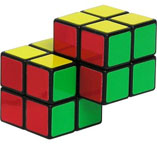Double 2x2 Cube \$9.95 A new twist on on a classic puzzle - a pair of connected 2x2 cubes. On a scale of 1-10, this puzzle rates an 8 for difficulty. 2 1/8 in x 1 1/2 in x 1 1/2 in.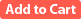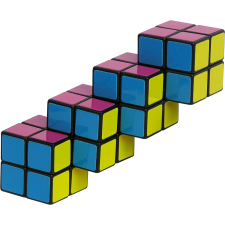Quadruple 2x2 Cube \$14.95 The object of this puzzle is to scramble all of the colors and then restore them to the starting position. This puzzle is extra tricky because it consists of four 2x2 cubes joined together in a row from corner to corner.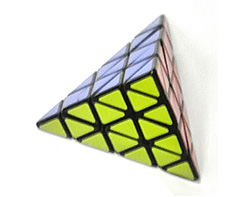Pyraminx Puzzle \$19.95 This "3 x 3" twisting puzzle is shaped like a regular tetrahedron, or triangular pyramid. Measures 3.7" x 3.2" x 3.2".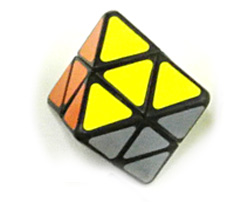Octahedron "2 x 2" Twisty Puzzle \$9.95 This "2 x 2" twisty puzzle is in the form of a regular octahedron (eight faces, each of which is an equilateral triangle).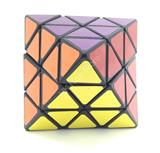Octahedron Twisty Puzzle \$14.95 This twisty puzzle is in the form of a regular octahedron (eight faces, each of which is an equilateral triangle).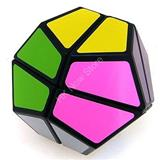Small Dodecahedron Twisty \$9.95 A simpler twisty puzzle based on the regular dodecahedron. This is a visually-appealing design, but relatively easy to solve.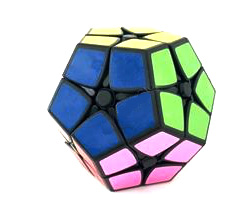Dodecahedron "2 x 2" Twisty Puzzle \$12.95 This "2 x 2" twisty puzzle is in the form of a regular dodecahedron.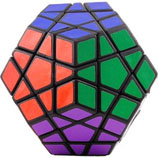Megaminx Dodecahedron Puzzle \$19.95 The 12 different colors of this high-quality rotational puzzle should throw you for a loop as to the solution. On a scale of 1-10, this puzzle rates a 10 for difficulty. 3 1/4 in x 3 1/4 in x 2 2/3 in.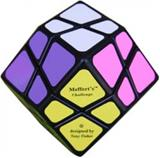Rhombic Dodecahedron Twisty Puzzle \$14.95 This twisty puzzle is in the form of a rhombic dodecahedron (twelve faces, each of which is a rhombus).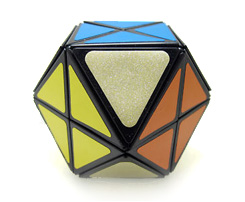Cuboctahedron Twisty Puzzle \$14.95 This "face-turning" twisty puzzle is in the form of a cuboctahedron (eight triangular faces and six square faces).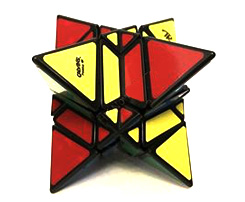Stella Octangula Twisty Puzzle \$19.95 This twisty puzzle is in the form of a stella octangula, which is same as two interpenetrating tetrahedra.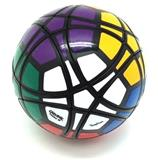Spherical Twisty Puzzle \$19.95 This spherical twisty puzzle has dodecahedral symmetry, with twelve colors.